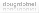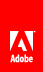# Finding substrings and patterns in strings

Flash Player 9 and later, Adobe AIR 1.0 and later

Substrings are sequential characters within a string. For example, the string "abc" has the following substrings: "" , "a" , "ab" , "abc" , "b" , "bc" , "c" . You can use ActionScript methods to locate substrings of a string.

Patterns are defined in ActionScript by strings or by regular expressions. For example, the following regular expression defines a specific pattern—the letters A, B, and C followed by a digit character (the forward slashes are regular expression delimiters):

`/ABC\d/`

ActionScript includes methods for finding patterns in strings and for replacing found matches with replacement substrings. These methods are described in the following sections.

Regular expressions can define intricate patterns. For more information, see Using regular expressions .

## Finding a substring by character position

The substr() and substring() methods are similar. Both return a substring of a string. Both take two parameters. In both methods, the first parameter is the position of the starting character in the given string. However, in the substr() method, the second parameter is the length of the substring to return, and in the substring() method, the second parameter is the position of the character at the end of the substring (which is not included in the returned string). This example shows the difference between these two methods:

```var str:String = "Hello from Paris, Texas!!!";
trace(str.substr(11,15)); // output: Paris, Texas!!!
trace(str.substring(11,15)); // output: Pari```

The slice() method functions similarly to the substring() method. When given two non-negative integers as parameters, it works exactly the same. However, the slice() method can take negative integers as parameters, in which case the character position is taken from the end of the string, as shown in the following example:

```var str:String = "Hello from Paris, Texas!!!";
trace(str.slice(11,15)); // output: Pari
trace(str.slice(-3,-1)); // output: !!
trace(str.slice(-3,26)); // output: !!!
trace(str.slice(-3,str.length)); // output: !!!
trace(str.slice(-8,-3)); // output: Texas```

You can combine non-negative and negative integers as the parameters of the slice() method.

## Finding the character position of a matching substring

You can use the indexOf() and lastIndexOf() methods to locate matching substrings within a string, as the following example shows:

```var str:String = "The moon, the stars, the sea, the land";
trace(str.indexOf("the")); // output: 10```

Notice that the indexOf() method is case-sensitive.

You can specify a second parameter to indicate the index position in the string from which to start the search, as follows:

```var str:String = "The moon, the stars, the sea, the land"
trace(str.indexOf("the", 11)); // output: 21```

The lastIndexOf() method finds the last occurrence of a substring in the string:

```var str:String = "The moon, the stars, the sea, the land"
trace(str.lastIndexOf("the")); // output: 30```

If you include a second parameter with the lastIndexOf() method, the search is conducted from that index position in the string working backward (from right to left):

```var str:String = "The moon, the stars, the sea, the land"
trace(str.lastIndexOf("the", 29)); // output: 21```

## Creating an array of substrings segmented by a delimiter

You can use the split() method to create an array of substrings, which is divided based on a delimiter. For example, you can segment a comma-delimited or tab-delimited string into multiple strings.

The following example shows how to split an array into substrings with the ampersand (&) character as the delimiter:

```var queryStr:String = "first=joe&last=cheng&title=manager&StartDate=3/6/65";
var params:Array = queryStr.split("&", 2); // params == ["first=joe","last=cheng"]```

The second parameter of the split() method, which is optional, defines the maximum size of the array that is returned.

You can also use a regular expression as the delimiter character:

```var str:String = "Give me\t5."
var a:Array = str.split(/\s+/); // a == ["Give","me","5."]```

For more information, see Using regular expressions and the ActionScript 3.0 Reference for the Adobe Flash Platform .

## Finding patterns in strings and replacing substrings

The String class includes the following methods for working with patterns in strings:

• Use the match() and search() methods to locate substrings that match a pattern.

• Use the replace() method to find substrings that match a pattern and replace them with a specified substring.

These methods are described in the following sections.

You can use strings or regular expressions to define patterns used in these methods. For more information on regular expressions, see Using regular expressions .### Trim Leading and Trailing 00s From a String

#### Finding matching substrings

The search() method returns the index position of the first substring that matches a given pattern, as shown in this example:

```var str:String = "The more the merrier.";
// (This search is case-sensitive.)
trace(str.search("the")); // output: 9 ```

You can also use regular expressions to define the pattern to match, as this example shows:

```var pattern:RegExp = /the/i;
var str:String = "The more the merrier.";
trace(str.search(pattern)); // 0```

The output of the trace() method is 0, because the first character in the string is index position 0. The i flag is set in the regular expression, so the search is not case-sensitive.

The search() method finds only one match and returns its starting index position, even if the g (global) flag is set in the regular expression.

The following example shows a more intricate regular expression, one that matches a string in double quotation marks:

```var pattern:RegExp = /"[^"]*"/;
var str:String = "The \"more\" the merrier.";
trace(str.search(pattern)); // output: 4

str = "The \"more the merrier.";
trace(str.search(pattern)); // output: -1
// (Indicates no match, since there is no closing double quotation mark.)```

The match() method works similarly. It searches for a matching substring. However, when you use the global flag in a regular expression pattern, as in the following example, match() returns an array of matching substrings:

```var str:String = "bob@example.com, omar@example.org";
var pattern:RegExp = /\w*@\w*\.[org|com]+/g;
var results:Array = str.match(pattern);```

The results array is set to the following:

`["bob@example.com","omar@example.org"]`

#### Replacing matched substrings

You can use the replace() method to search for a specified pattern in a string and replace matches with the specified replacement string, as the following example shows:

```var str:String = "She sells seashells by the seashore.";
var pattern:RegExp = /sh/gi;
trace(str.replace(pattern, "sch")); //sche sells seaschells by the seaschore. ```

Note that in this example, the matched strings are not case-sensitive because the i ( ignoreCase ) flag is set in the regular expression, and multiple matches are replaced because the g ( global ) flag is set. For more information, see Using regular expressions .

You can include the following \$ replacement codes in the replacement string. The replacement text shown in the following table is inserted in place of the \$ replacement code:

\$ Code

Replacement Text

\$\$

\$

\$&

The matched substring.

\$`

The portion of the string that precedes the matched substring. This code uses the straight left single quotation mark character ( ` ), not the straight single quotation mark ( ' ) or the left curly single quotation mark ( ' ).

\$'

The portion of the string that follows the matched substring. This code uses the straight single quotation mark ( ' ).

\$ n

The n th captured parenthetical group match, where n is a single digit, 1-9, and \$n is not followed by a decimal digit.

\$ nn

The nn th captured parenthetical group match, where nn is a two-digit decimal number, 01–99. If the nn th capture is undefined, the replacement text is an empty string.

For example, the following shows the use of the \$2 and \$1 replacement codes, which represent the first and second capturing group matched:

```var str:String = "flip-flop";
var pattern:RegExp = /(\w+)-(\w+)/g;
trace(str.replace(pattern, "\$2-\$1")); // flop-flip```

You can also use a function as the second parameter of the replace() method. The matching text is replaced by the returned value of the function.

```var str:String = "Now only \$9.95!";
var price:RegExp = /\\$([\d,]+.\d+)+/i;
trace(str.replace(price, usdToEuro));

function usdToEuro(matchedSubstring:String,                                  capturedMatch1:String,                                  index:int,                                  str:String):String
{
var usd:String = capturedMatch1;
usd = usd.replace(",", "");
var exchangeRate:Number = 0.853690;
var euro:Number = parseFloat(usd) * exchangeRate;
const euroSymbol:String = String.fromCharCode(8364);
return euro.toFixed(2) + " " + euroSymbol;
}```

When you use a function as the second parameter of the replace() method, the following arguments are passed to the function:

• The matching portion of the string.

• Any capturing parenthetical group matches. The number of arguments passed this way will vary depending on the number of parenthetical matches. You can determine the number of parenthetical matches by checking arguments.length - 3 within the function code.

• The index position in the string where the match begins.

• The complete string.// Ethnio survey code removed